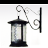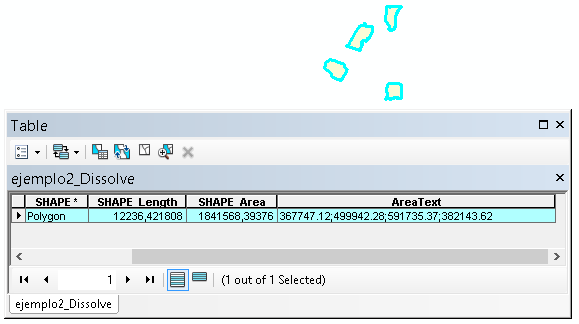# Multipart polygon - individual part areas

1437
9
09-10-2017 11:45 PMOccasional Contributor

Good day,

I have a polygon feature class in a file geodatabase. There are a few multipart polygons in the dataset. I have added 2 fields to the dataset to store the number of parts (numeric) and one to store a list of the individual part areas (string).

I have populated the field with the number of parts using one of the functions in the EasyCalculate Add-In. However, I require assistance with populating my string field with a delimited list of the individual part areas for any multipart polygons in the dataset. So for example, if a polygon has 4 parts to it, the value for the string field that I want calculated should look like this -> "541.00;236.54;147.85;391.25" being the individual areas of the parts of the multipart polygon.

I found some python code that iterated through the polygons and their respective parts and printed the results to the python window. However I need to concatenate the areas into a delimited string and write the string to the feature. I cannot seem to get that part of the code correct. Here is the link to the thread on another website for accessing the part areas python 2.7 - Getting area of multipart features using SearchCursor() of ArcPy? - Geographic Informat...

Please could someone help with the code to write the individual areas in the string back to the feature?

TIA

Tags (2)
1 Solution

Accepted SolutionsbyEsri Esteemed Contributor

Hi Alan Tonkin ,

In theory one should be able to do this using the following code:

``````def PolygonAreaText(polygon):
lst_area = []
for part in polygon:
lst_pnts = []
for pnt in part:
lst_pnts.append(pnt)
pol2 = arcpy.Polygon(arcpy.Array(lst_pnts), polygon.spatialReference)
if pol2.area > 0:
lst_area.append(str(round(pol2.area, 2)))
return ';'.join(lst_area)‍‍‍‍‍‍‍‍‍‍``````

However, when I ran the code on an example multipart polygon dataset it bounced an error. Probably because the polygon is passed to the function as a GPVARIANT and doesn't allow iteration.

The alternative is to create a script that will loop through the features and updates the field that will hold the area of the parts:

``````def main():
import arcpy
fc = r'C:\Users\xbakker\Documents\ArcGIS\Default.gdb\ejemplo2_Dissolve'
fld_areatext = 'AreaText'

flds = ('SHAPE@', fld_areatext)
with arcpy.da.UpdateCursor(fc, flds) as curs:
for row in curs:
polygon = row
area_text = PolygonAreaText(polygon)
print area_text
row = area_text
curs.updateRow(row)

def PolygonAreaText(polygon):
lst_area = []
for part in polygon:
lst_pnts = []
for pnt in part:
lst_pnts.append(pnt)
pol2 = arcpy.Polygon(arcpy.Array(lst_pnts), polygon.spatialReference)
if pol2.area > 0:
lst_area.append(str(round(pol2.area, 2)))
return ';'.join(lst_area)

if __name__ == '__main__':
main()‍‍‍‍‍‍‍‍‍‍‍‍‍‍‍‍‍‍‍‍‍‍‍‍‍‍‍``````

In this case you would have to change the path to your featureclass on line 3 and the name of the field that will hold the areas of the parts. Below the result on my simple dataset:9 RepliesbyEsri Esteemed Contributor

Hi Alan Tonkin ,

In theory one should be able to do this using the following code:

``````def PolygonAreaText(polygon):
lst_area = []
for part in polygon:
lst_pnts = []
for pnt in part:
lst_pnts.append(pnt)
pol2 = arcpy.Polygon(arcpy.Array(lst_pnts), polygon.spatialReference)
if pol2.area > 0:
lst_area.append(str(round(pol2.area, 2)))
return ';'.join(lst_area)‍‍‍‍‍‍‍‍‍‍``````

However, when I ran the code on an example multipart polygon dataset it bounced an error. Probably because the polygon is passed to the function as a GPVARIANT and doesn't allow iteration.

The alternative is to create a script that will loop through the features and updates the field that will hold the area of the parts:

``````def main():
import arcpy
fc = r'C:\Users\xbakker\Documents\ArcGIS\Default.gdb\ejemplo2_Dissolve'
fld_areatext = 'AreaText'

flds = ('SHAPE@', fld_areatext)
with arcpy.da.UpdateCursor(fc, flds) as curs:
for row in curs:
polygon = row
area_text = PolygonAreaText(polygon)
print area_text
row = area_text
curs.updateRow(row)

def PolygonAreaText(polygon):
lst_area = []
for part in polygon:
lst_pnts = []
for pnt in part:
lst_pnts.append(pnt)
pol2 = arcpy.Polygon(arcpy.Array(lst_pnts), polygon.spatialReference)
if pol2.area > 0:
lst_area.append(str(round(pol2.area, 2)))
return ';'.join(lst_area)

if __name__ == '__main__':
main()‍‍‍‍‍‍‍‍‍‍‍‍‍‍‍‍‍‍‍‍‍‍‍‍‍‍‍``````

In this case you would have to change the path to your featureclass on line 3 and the name of the field that will hold the areas of the parts. Below the result on my simple dataset:byMVP Esteemed Contributor

xander_bakker‌, I am a bit confused, not about the overall solution but your first comment.  You said the first code block should work but doesn't, but then the code block was used as is in your second code block.  Was there a different code block that generated the error you speak of?byEsri Esteemed Contributor

Hi Joshua Bixby ,

Sorry for the confusion. Indeed the code block works in the stand alone script, but when used as function in the Field Calculator it throws an error.Occasional Contributor

Hi Xander,

Thank you for your reply to my thread. I am running this from the Python window within my ArcMap MXD so I have taken some guidelines from your code posted above.

This is what it looks like now and it is working 100% as I need it to:

import arcpy
... from arcpy import env
... env.workspace = 'some.gdb'
... fc = 'someFC'
... fld_areatext = 'AREA_TEXT'
... flds = ('SHAPE@', fld_areatext)
... edit = arcpy.da.Editor(env.workspace)
... edit.startEditing(False, True)
... edit.startOperation()
... with arcpy.da.UpdateCursor(fc, flds) as curs:
...     for row in curs:
...         polygon = row
...         lst_area = []
...         for part in polygon:
...             poly = arcpy.Polygon(part)
...             lst_area.append(round(poly.area,2))
...         poly_text = ';'.join(lst_area)
...         row = poly_text
...         curs.updateRow(row)
... edit.stopOperation()
... edit.stopEditing(True)

Many thanks for your assistance. Much appreciated!byEsri Esteemed Contributor

Great to hear that it is working!Occasional Contributor

Hi Xander,

Since you were so helpful with this thread, maybe you could suggest whether or not the following would be possible without creating additional datasets to accomplish what I need:

Let's say I have a multipart polygon feature that has 4 parts to it. I would like a way to label each individual part with its part number (1 to 4) and its corresponding area. Is there any way of accomplishing this without making interim/temporary datasets?byEsri Esteemed Contributor

I'm afraid that will not be possible since labels are based on attributes and the parts are all part of a single feature. You will have to apply multi part to single part tool (Multipart To Singlepart—Help | ArcGIS Desktop ) to achieve that result.Occasional Contributor

I had a feeling that you were going to say that. I wanted to avoid creating disjointed datasets to achieve the labelling that I require.byEsri Esteemed Contributor

Is it really necessary to maintain the dataset with multipart features or could this be changed to single parts? You could also create label point for each part and label those, but this will include the creation of a new dataset.